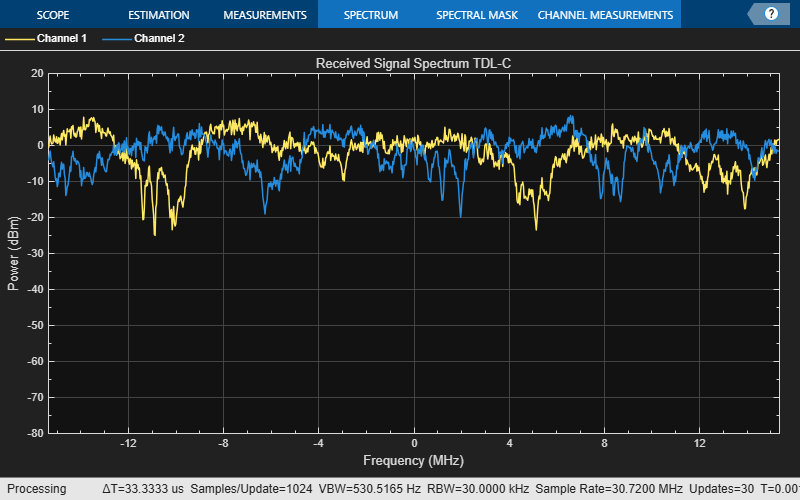Documentation

This is machine translation

Mouseover text to see original. Click the button below to return to the English version of the page.

Transmission over MIMO Channel Model with Delay Profile TDL

Display waveform spectrum received through a Tapped Delay Line (TDL) multi-input/multi-output (MIMO) channel model from TR 38.901 Section 7.7.2 using an `nrTDLChannel` System object.

Define the channel configuration structure using an `nrTDLChannel` System object. Use delay profile TDL-C from TR 38.901 Section 7.7.2, a delay spread of 300 ns, and UT velocity of 30 km/h:

```v = 30.0; % UT velocity in km/h fc = 4e9; % carrier frequency in Hz c = physconst('lightspeed'); % speed of light in m/s fd = (v*1000/3600)/c*fc; % UT max Doppler frequency in Hz tdl = nrTDLChannel; tdl.DelayProfile = 'TDL-C'; tdl.DelaySpread = 300e-9; tdl.MaximumDopplerShift = fd;```

Create a random waveform of 1 subframe duration with 1 antenna.

```SR = 30.72e6; T = SR * 1e-3; tdl.SampleRate = SR; tdlinfo = info(tdl); Nt = tdlinfo.NumTransmitAntennas; txWaveform = complex(randn(T,Nt),randn(T,Nt));```

Transmit the input waveform through the channel.

`rxWaveform = tdl(txWaveform);`

```analyzer = dsp.SpectrumAnalyzer('SampleRate',tdl.SampleRate); analyzer.Title = ['Received Signal Spectrum ' tdl.DelayProfile]; analyzer(rxWaveform);```